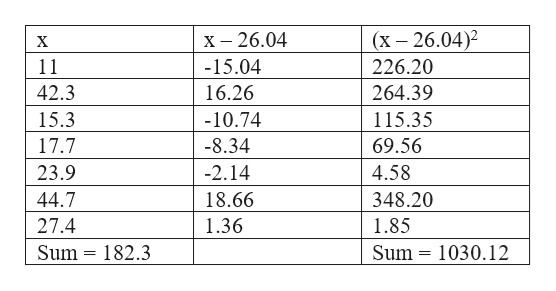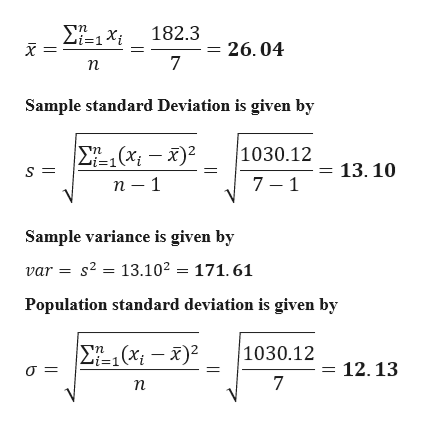# This data is from a sample. Calculate the mean, standard deviation, and variance.x1142.315.317.723.944.727.4Please show the following answers to 2 decimal places.Sample Mean =Sample Standard Deviation =Sample Variance = (Please use the standard deviation above for your calculation.)Ooops - now you discover that the data was actually from a population! So now you must give the population standard deviation.Population Standard Deviation =

Question
4 views

This data is from a sample. Calculate the mean, standard deviation, and variance.

x
11
42.3
15.3
17.7
23.9
44.7
27.4

Sample Mean =

Sample Standard Deviation =

Sample Variance = (Please use the standard deviation above for your calculation.)

Ooops - now you discover that the data was actually from a population! So now you must give the population standard deviation.

Population Standard Deviation =

check_circle

Step 1

Given data and calculation for mean and standard deviation is shown belowhelp_outlineImage Transcriptionclose(x – 26.04)2 х - 26.04 11 -15.04 226.20 42.3 16.26 264.39 -10.74 15.3 115.35 17.7 -8.34 69.56 -2.14 23.9 4.58 18.66 44.7 348.20 27.4 1.36 1.85 Sum = 182.3 Sum = 1030.12 fullscreen
Step 2

Mean is given by

...help_outlineImage TranscriptioncloseΣ-1Χ 182.3 26.04 Sample standard Deviation is given by 1030.12 E (xi – x)² %3D1 13. 10 п — 1 Sample variance is given by var = s2 = 13.10² = 171. 61 Population standard deviation is given by E-,(x; – x)2 1030.12 in 12.13 п fullscreen

### Want to see the full answer?

See Solution

#### Want to see this answer and more?

Solutions are written by subject experts who are available 24/7. Questions are typically answered within 1 hour.*

See Solution
*Response times may vary by subject and question.
Tagged in

### Data